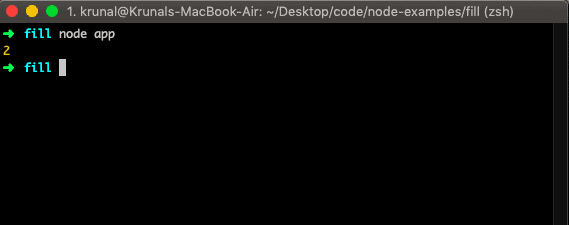# JavaScript findIndex: The Complete Guide

0
210ES2015 added a new method called findIndex() to the Array.prototype, which lets you find the first element in the array that satisfies a provided testing function. The findIndex() method in JavaScript executes a function once for each item in an array.

## JavaScript findIndex

JavaScript array findIndex() is a built-in function that returns an index of the first item in an array that satisfies the provided callback function; otherwise, it returns -1, indicating no element passed the test. The findIndex() function accepts two arguments which are function and thisValue(optional).

The findIndex() method executes the function once for each item present in the Array: If it finds the array element where the function returns the true value, the findIndex() method returns the index of that array element (and does not check the remaining values).

### Syntax

The syntax is the following.

`array.findIndex(function(currentValue, index, arr), thisValue)`

### Parameters

The function is the required argument. It will run for every item in an array.

The currentValue is the required parameter. It is the value of the current item.

The index is an optional parameter. It is an array index of the current element.

The arr is an optional parameter. It is the current array object.

The thisValue is an optional parameter. The value to be passed to a function to be used as its “this” value.

### Example

Now, let us take a simple example.

```// app.js

let ages = [3, 10, 18, 20];

console.log(ages.findIndex(age => age > 10));
```

In the above example, we have defined an array and the call the findIndex() function on that Array.

Pass the arrow function, which checks if the item in the Array is higher than 10. If the item value > 10 is found, it will log that item’s index. Then, run that file by typing the node app on the terminal.So the first item, higher than 10, is 18, and its index is 2. So 2 is logged on the console.

The findIndex() method will return the index based on the written logic inside the given function. The main difference between findIndex() and indexOf() is that we need to provide the element to the indexOf() function. In contrast, on the other side, the findIndex() method expects a function that has defined the logic on which we get the index.

## Javascript findIndex() vs find()

The main difference between find() and findIndex() is that find() method returns an item value, but the findIndex() method returns the element index.

```// app.js

let arr = [11, 21, 19, 29, 18, 10, 46];

data = arr.find((v) => {
return v % 2 === 0;
});

console.log(data)```

#### Output

```node app
18```

An Array.find() method calls the `(v)=>{ return v % 2 === 0;}` predicate for each element in the Array until the predicate returns true. In this case, the element is returned. If no element is found, undefined is returned.

```// app.js

let arr = [11, 21, 19, 29, 17, 23, 31];

data = arr.find((v) => {
return v % 2 === 0;
});

console.log(data)```

#### Output

```node app
undefined```

The findIndex() method is quite similar to the find() method but returns the element’s index instead of the element itself.

It takes a predicate and returns the index of the first element in the array that satisfies the predicate. If no element exists, -1 is returned.

See the following code.

```// app.js

let arr = [11, 21, 19, 29, 17, 23, 31];

index = arr.findIndex((v) => {
return v % 2 === 0;
});

console.log(index)```

#### Output

```node app
-1```

You can see that the index is -1 because there is no satisfying element in the Array. There is no even element in the Array. That is why the function returns the -1 index.

Now, let’s write an example where the condition is satisfied with an element.

```// app.js

let arr = [11, 21, 19, 20, 17, 24, 31];

index = arr.findIndex((v) => {
return v % 2 === 0;
});

console.log(index)```

In the above code, we have defined an array with even elements. There are two even elements in the Array whose index is 3 and 5. Now, let’s run the program and see the output.

```node app
3```

That means it immediately returns the index if it finds the index. The remaining even element’s index won’t be returning in this case.

## Conclusion

The findIndex() does not execute the function for array elements without values. Therefore, the findIndex() does not change the original Array.

The findIndex() method executes the callback function once for every array index 0..length-1 (inclusive) in an array until it finds one where the provided function returns a true value (a value that coerces to true).

That’s it for this tutorial.